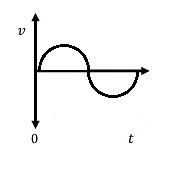These calculators are used to calculate rotary motion. If you need to calculate linear motion, please use the linear motion calculators.
Unit Converter
Conversion Type Units Units
$$T = J \times \alpha$$

Torque = Inertia x Angular Acceleration

To size a stage properly the torque must be known. If torque is not known it must be calculated from this equation. The inertia is the total inertia of the customer payload plus the inertia of the moving components of the stage. If the acceleration component is not known it must be calculated. Calculators are provided under the Acceleration tab for estimating the acceleration of a system. Once the torque is determined, the duty cycle for all of the specific torques must be determined to calculate the RMS force, which is the average required torque. The Torque RMS tab provides the tools needed to determine the RMS Torque of a motion profile.

Variable Description Units Calculation
J Inertia
α Acceleration (radians/s²)
T Torque 0
*accelerations up to 10,000 radians/s2 are possible$$\Large \alpha = \frac{4 \times \theta }{t^2}$$
Variable Description Units Calculation
θ Angular Displacement
t Time to complete move (sec)
α Acceleration (radians/s²) 0
*accelerations up to 10,000 radians/s2 are possible$$\Large \alpha = 2\pi^2 f^2 \theta$$
Known Variables
Frequency and Displacement are known
Velocity and Frequency are known
Displacement and Velocity are known

Variable Description Units Calculation
θ Angular Displacement
f Frequency (Hz)
v Velocity
α Acceleration (radian/s²) 0
*accelerations up to 10,000 radians/s2 are possible
$$\Large T_{rms} = \sqrt{\left ( T_{a} \right )^2 \times t_{a} + \left ( T_{c} \right )^2 \times t_{c} + \left ( T_{d} \right )^2 \times t_{d} \over \left ( t_{on} + t_{off} \right )}$$
Variable Description Units Calculation
Tα Acceleration Torque
Tc Constant Velocity Torque
Td Deceleration Torque
ta Time to accelerate (sec)
tc Time at constant velocity (sec)
td Time to decelerate (sec)
ton ta + tc + td (sec)
toff Dwell time (sec)
Trms Average required torque 0
$$\Large Duty Cycle\left ( \% \right ) ={ t_{on} \over \left ( t_{on} + t_{off}\right )} \times 100$$
Variable Description Calculation
ton Time with power applied (sec)
toff Dwell time (sec)
Duty Cycle (%) Percentage of total time spent active 0
Example
Duty Cycle = 1 sec on, 3 sec off

Duty Cycle = 1/(1+3) = 1/4

Duty Cycle = 25%
Note: Duty Cycle is only for DC motors.
$$\Large T@100\% = Torque At DutyCycle \div \sqrt{ 1 \over DutyCycle}$$
Variable Calculation
Duty Cycle %
Torque at Duty Cycle
Torque at 100 % 0
Example
Torque at 10% Duty Cycle = 1 N-m X (1/10%)1/2

Torque at 10% Duty Cycle = 1 N-m X 3.16

Torque at 10% Duty Cycle = 3.16 N-m
Note: This calculation is only for DC motors.
Use the following formula for AC motors:
AC Duty Cycle Calculations
Torque @ 50% = Torque @ 100% * 1.75
v@ 15% = Torque @ 100% * 5
Torque @ 3% = Torque @ 100% * 8